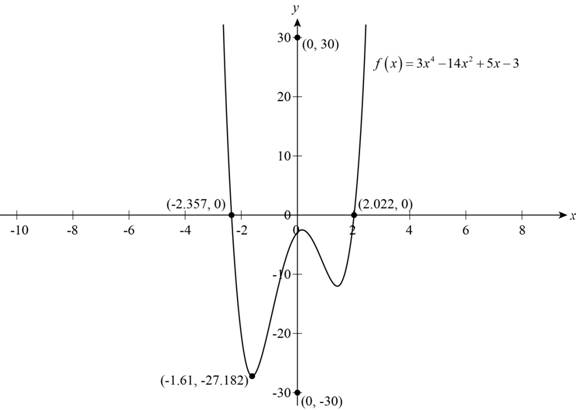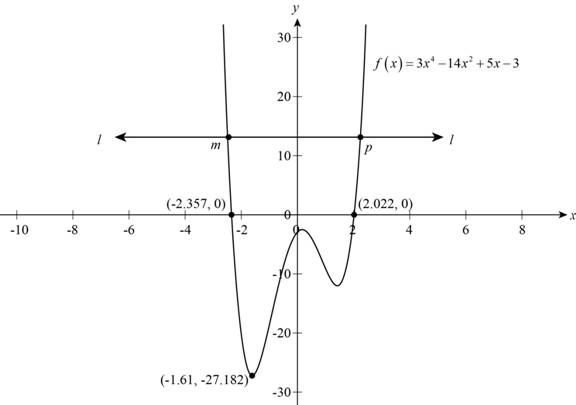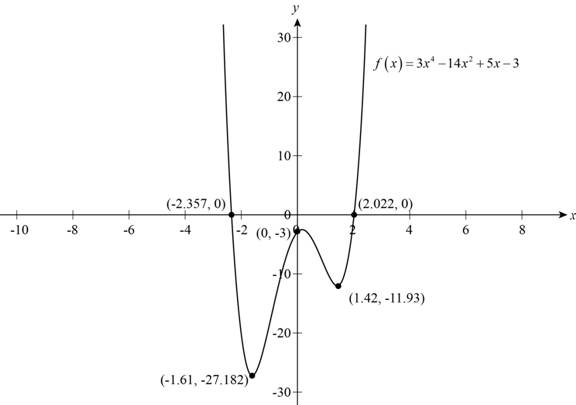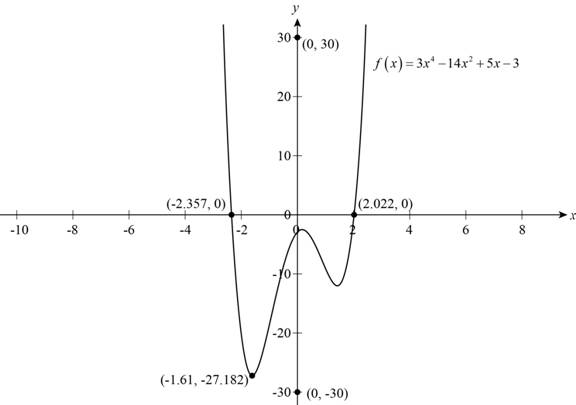# The graph of f ( x ) = 3 x 4 − 14 x 2 + 5 x − 3 in an appropriate viewing rectangle.### Precalculus: Mathematics for Calcu...

6th Edition
Stewart + 5 others
Publisher: Cengage Learning
ISBN: 9780840068071### Precalculus: Mathematics for Calcu...

6th Edition
Stewart + 5 others
Publisher: Cengage Learning
ISBN: 9780840068071

#### Solutions

Chapter 2, Problem 12T

(a)

To determine

## To sketch: The graph of f(x)=3x4−14x2+5x−3 in an appropriate viewing rectangle.

Expert Solution

### Explanation of Solution

The domain of the given function is (,) and range is (27.182,) so appropriate viewing rectangle must be larger than range so, y coordinate of appropriate viewing rectangle is [30,30] .

Sketch the graph of given function as shown below.Figure (1)

Above figure shows the graph of function f(x)=3x414x2+5x3 .

(b)

To determine

### To find: The function f(x)=3x4−14x2+5x−3 is one-to-one or not.

Expert Solution

The function f(x)=3x414x2+5x3 is not one to one.

### Explanation of Solution

To find whether the function is one-to-one or not, draw a horizontal line on the graph of function if it intersects it at more than one point than it is not one-to-one.

Draw the horizontal line on graph of the given function as shown below.Figure (2)

Observe from Figure (2) that horizontal line l-l intersects the graph of given function at two points m and p.

Thus, the function is not one-to-one.

(c)

To determine

### To find: The local maximum and local minimum value of the given function f(x)=3x4−14x2+5x−3 from the graph up to two decimal place.

Expert Solution

Local minimum values are 27.18at-1.61 and 11.93at1.42 and local maximum value is 3at0 .

### Explanation of Solution

The graph of given function is shown below.Figure (3)

Any Point a on any curve is called local maximum when f(a)f(x) , here x is a point near to point a.

Any point a on any curve is called local minimum when f(a)f(x) , here x is a point near to point a.

Observe from Figure (3) that point a and b are local minimum and point c is local minimum.

Coordinates of point a,b,c are (1.61,27.18),(1.42,11.93),(0,3) .

Thus local minimum values are 27.18at-1.61 and 11.93at1.42 and local maximum value is 3at0 .

(d)

To determine

### To find: The range of the function f(x)=3x4−14x2+5x−3 from the graph.

Expert Solution

The range of given function in set notation is (27.182,) .

### Explanation of Solution

The graph of given function is shown below.Figure (4)

The minimum value of dependent variable as shown in Figure (4) is 27.182 and maximum value is extended up to infinity.

So, the range of given function in set notation is (27.182,) .

(e)

To determine

### To find: The intervals on which function is increasing and decreasing.

Expert Solution

Function is increasing in interval (1.61,0)(1.42,) and function is decreasing in interval (,27.18)(0,1.42) .

### Explanation of Solution

The graph of given function is shown below.Figure (5)

The function is said to be increasing when its graph is rising and function is said to be decreasing when its graph is falling.

Observe from the graph that it is falling in interval (,27.18) and (0,1.42) and graph is rising in (1.61,0) and (1.42,) .

Thus, the function is increasing in (1.61,0)(1.42,) and function is decreasing in (,27.18)(0,1.42) .

### Have a homework question?

Subscribe to bartleby learn! Ask subject matter experts 30 homework questions each month. Plus, you’ll have access to millions of step-by-step textbook answers!